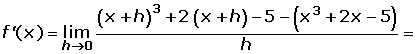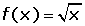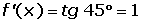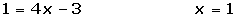## Exercise 1at x = -5.

## Exercise 2at x = 1.

## Exercise 3at x = 2.

## Exercise 4at x = 3.

## Exercise 5at x = 2.

## Exercise 6

Given the equation f(x) = 2x² − 3x − 1, find the coordinates of the point on the curve in which the tangent forms a 45° angle with the x-axis.

## Solution of exercise 1

Find the value of the derivativeat x = 5.## Solution of exercise 2

Find the value of the derivativeat x = 1.## Solution of exercise 3

Find the value of the derivativeat x = 2.## Solution of exercise 4

Find the value of the derivativeat x = 3.## Solution of exercise 5

Find the value of the derivativeat x = 2.## Solution of exercise 6

Given the equation f(x) = 2x² − 3x − 1, find the coordinates of the point on the curve in which the tangent forms a 45° angle with the x-axis .Did you like the article?(1 votes, average: 5.00 out of 5)Loading...

Emma

I am passionate about travelling and currently live and work in Paris. I like to spend my time reading, gardening, running, learning languages and exploring new places.

Did you like
this resource?

Bravo!